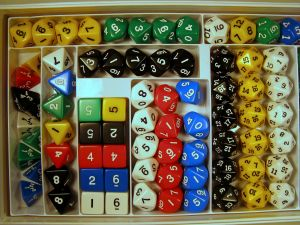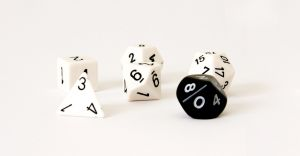# 9.1 - The Basic Idea

9.1 - The Basic Idea

Every time we perform a hypothesis test, this is the basic procedure that we will follow:

1. We'll make an initial assumption about the population parameter.
2. We'll collect evidence or else use somebody else's evidence (in either case, our evidence will come in the form of data).
3. Based on the available evidence (data), we'll decide whether to "reject" or "not reject" our initial assumption.

Let's try to make this outlined procedure more concrete by taking a look at the following example.

## Example 9-1A four-sided (tetrahedral) die is tossed 1000 times, and 290 fours are observed. Is there evidence to conclude that the die is biased, that is, say, that more fours than expected are observed?

As the basic hypothesis testing procedure outlines above, the first step involves stating an initial assumption. It is:

Assume the die is unbiased. If the die is unbiased, then each side (1, 2, 3, and 4) is equally likely. So, we'll assume that p, the probability of getting a 4 is 0.25.

In general, the initial assumption is called the null hypothesis, and is denoted $$H_0$$. (That's a zero in the subscript for "null"). In statistical notation, we write the initial assumption as:

$$H_0 \colon p=0.25$$

That is, the initial assumption involves making a statement about a population proportion.

Now, the second step tells us that we need to collect evidence (data) for or against our initial assumption. In this case, that's already been done for us. We were told that the die was tossed $$n=1000$$ times, and $$y=290$$ fours were observed. Using statistical notation again, we write the collected evidence as a sample proportion:

$$\hat{p}=\dfrac{y}{n}=\dfrac{290}{1000}=0.29$$

Now we just need to complete the third step of making the decision about whether or not to reject our initial assumption that the population proportion is 0.25. Recall that the Central Limit Theorem tells us that the sample proportion:

$$\hat{p}=\dfrac{Y}{n}$$

is approximately normally distributed with (assumed) mean:

$$p_0=0.25$$

and (assumed) standard deviation:

$$\sqrt{\dfrac{p_0(1-p_0)}{n}}=\sqrt{\dfrac{0.25(0.75)}{1000}}=0.01369$$

That means that:

$$Z=\dfrac{\hat{p}-p_0}{\sqrt{\dfrac{p_0(1-p_0)}{n}}}$$

follows a standard normal $$N(0,1)$$ distribution. So, we can "translate" our observed sample proportion of 0.290 onto the $$Z$$ scale. Here's a picture that summarizes the situation:

So, we are assuming that the population proportion is 0.25 (in blue), but we've observed a sample proportion 0.290 (in red) that falls way out in the right tail of the normal distribution. It certainly doesn't appear impossible to obtain a sample proportion of 0.29. But, that's what we're left with deciding. That is, we have to decide if a sample proportion of 0.290 is more extreme that we'd expect if the population proportion $$p$$ does indeed equal 0.25.

There are two approaches to making the decision:

1. one is called the "critical value" (or "critical region" or "rejection region") approach
2. and the other is called the "$$p$$-value" approach

Until we get to the page in this lesson titled The $$p$$-value Approach, we'll use the critical value approach.

###Example (continued)

A four-sided (tetrahedral) die is tossed 1000 times, and 290 fours are observed. Is there evidence to conclude that the die is biased, that is, say, that more fours than expected are observed?

Okay, so now let's think about it. We probably wouldn't reject our initial assumption that the population proportion $$p=0.25$$ if our observed sample proportion were 0.255. And, we might still not be inclined to reject our initial assumption that the population proportion $$p=0.25$$ if our observed sample proportion were 0.27. On the other hand, we would almost certainly want to reject our initial assumption that the population proportion $$p=0.25$$ if our observed sample proportion were 0.35. That suggests, then, that there is some "threshold" value that once we "cross" the threshold value, we are inclined to reject our initial assumption. That is the critical value approach in a nutshell. That is, critical value approach tells us to define a threshold value, called a "critical value" so that if our "test statistic" is more extreme than the critical value, then we reject the null hypothesis.

Let's suppose that we decide to reject the null hypothesis $$H_0:p=0.25$$ in favor of the "alternative hypothesis" $$H_A \colon p>0.25$$ if:

$$\hat{p}>0.273$$ or equivalently if $$Z>1.645$$

Here's a picture of such a "critical region" (or "rejection region"):

Note, by the way, that the "size" of the critical region is 0.05. This will become apparent in a bit when we talk below about the possible errors that we can make whenever we conduct a hypothesis test.

At any rate, let's get back to deciding whether our particular sample proportion appears to be too extreme. Well, it looks like we should reject the null hypothesis (our initial assumption $$p=0.25$$) because:

$$\hat{p}=0.29>0.273$$

or equivalently since our test statistic:

$$Z=\dfrac{\hat{p}-p_0}{\sqrt{\dfrac{p_0(1-p_0)}{n}}}=\dfrac{0.29-0.25}{\sqrt{\dfrac{0.25(0.75)}{1000}}}=2.92$$

is greater than 1.645.

Our conclusion: we say there is sufficient evidence to conclude $$H_A:p>0.25$$, that is, that the die is biased.

By the way, this example involves what is called a one-tailed test, or more specifically, a right-tailed test, because the critical region falls in only one of the two tails of the normal distribution, namely the right tail.

Before we continue on the next page at looking at two more examples, let's revisit the basic hypothesis testing procedure that we outlined above. This time, though, let's state the procedure in terms of performing a hypothesis test for a population proportion using the critical value approach. The basic procedure is:

1. State the null hypothesis $$H_0$$ and the alternative hypothesis $$H_A$$. (By the way, some textbooks, including ours, use the notation $$H_1$$ instead of $$H_A$$ to denote the alternative hypothesis.)
2. Calculate the test statistic:
3. $$Z=\dfrac{\hat{p}-p_0}{\sqrt{\dfrac{p_0(1-p_0)}{n}}}$$

4. Determine the critical region.
5. Make a decision. Determine if the test statistic falls in the critical region. If it does, reject the null hypothesis. If it does not, do not reject the null hypothesis.

Now, back to those possible errors we can make when conducting such a hypothesis test.

## Possible Errors

So, argh! Every time we conduct a hypothesis test, we have a chance of making an error. (Oh dear, why couldn't I have chosen a different profession?!)

1. If we reject the null hypothesis $$H_0$$ (in favor of the alternative hypothesis $$H_A$$) when the null hypothesis is in fact true, we say we've committed a Type I error. For our example above, we set P(Type I error) equal to 0.05:

Aha! That's why the 0.05! We wanted to minimize our chance of making a Type I error! In general, we denote $$\alpha=P(\text{Type I error})=$$ the "significance level of the test." Obviously, we want to minimize $$\alpha$$. Therefore, typical $$\alpha$$ values are 0.01, 0.05, and 0.10.

2. If we fail to reject the null hypothesis when the null hypothesis is false, we say we've committed a Type II error. For our example, suppose (unknown to us) that the population proportion $$p$$ is actually 0.27. Then, the probability of a Type II error, in this case, is:

$$P(\text{Type II Error})=P(\hat{p}<0.273\quad if \quad p=0.27)=P\left(Z<\dfrac{0.273-0.27}{\sqrt{\dfrac{0.27(0.73)}{1000}}}\right)=P(Z<0.214)=0.5847$$

In general, we denote $$\beta=P(\text{Type II error})$$. Just as we want to minimize $$\alpha=P(\text{Type I error})$$, we want to minimize $$\beta=P(\text{Type II error})$$. Typical $$\beta$$ values are 0.05, 0.10, and 0.20.

  Link ↥ Has Tooltip/Popover Toggleable Visibility